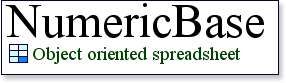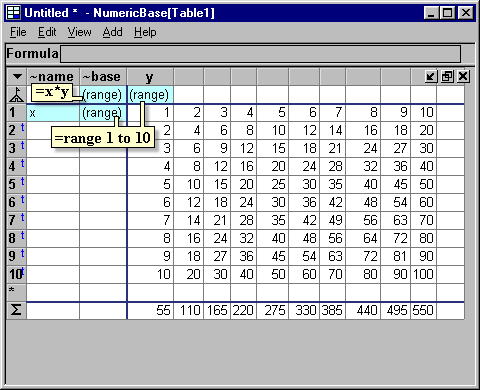# Multiplication table

Multiplication table
This example shows a multiplication table that is implemented with only three formulas -two range formulas, and one calculation formula =x*y.Screenshot: multiplication table

Source code
Following is the source code for this example. To use it in your copy of NumericBase, copy and paste it in the View Source window.

table Table1{    ~name:=!    ~base:=x*y!    y:=range 1 to 10!    <1>:={~name:="x",~base:=range 1 to 10,} } maximized result(Table1);
Listing: source code for the multiplication table example.

Changing the solution
You can change the multiplication table by changing the range formulas or the calculation formula. The following animated screenshot shows and example: we change the multiplication table to an "addition table" by changing one formula:Animated screenshot: changing from multiplication to addition